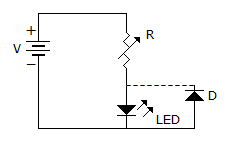Electronics and Communication Engineering - Electronic Devices and Circuits

6.

In p-n-p transistor the current IE has two components viz. IEP due to injection of holes from p-region to n-region and IE due to injection of electrons from n-region to p-region. Then

 A. IEp and IEn are almost equal B. IEp >> IEn C. IEn >> IEp D. either (a) or (c)

Explanation:

Emitter is p-type in p-n-p transistor.

Therefore holes are majority carriers.

7.

In an n channel JFET, the gate is

 A. n type B. p type C. either n or p D. partially n & partially p

Explanation:

Since channel is n type gate must be p type.

8.

The amount of photoelectric emission current depends on

 A. frequency of incident radiation B. intensity of incident radiation C. both frequency and intensity of incident radiation D. none of the above

Explanation:

Only the intensity of incident radiation governs the amount of photoelectric emission.

9.

Assertion (A): A p-n junction has high resistance in reverse direction.

Reason (R): When a reverse bias is applied to p-n junction, the width of depletion layer increases.

 A. Both A and R are true and R is correct explanation of A B. Both A and R are true but R is not a correct explanation of A C. A is true but R is false D. A is false but R is true

Explanation:

The increase in reverse resistance is due to widening of depletion layer.

10.

In the circuit of figure the function of resistor R and diode D areA. to limit the current and to protect LED against over voltage B. to limit the voltage and to protect LED against over current C. to limit the current and protect LED against reverse breakdown voltage. D. none of the above.